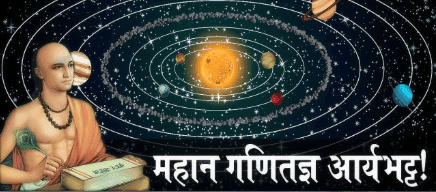Home » » Mathematician Aryabhatta pratham

# Mathematician Aryabhatta pratham

## 1.Biography of Aryabhatta pratham

Aryabhata (first) was born in 476 AD in Kusumpur town near Patna. Three of his texts are known - Demographics, Aryabhatiya and Tantra.Among them Aryabhatiya is the most famous book. Other astrological names of this name came to be around 950 AD, who composed a book called 'Maha-Siddhant'. So call them Aryabhatta first.## (2,) Aryabhata's contribution to mathematics (Contribution of Aryabhatta in Mathematics)-

He compiled his book 'Aryabhatiya' in Kusumpur district in 499 AD. This district is called Patna nowadays. There are 121 stanzas in Aryabhatiya, which are divided into four sections - (1.) Geet Patika (2.) Mathematical Foot (3.) Kalikriapad and (4) Golapad.
Golapad is very small text.Golapple is very small text. There are 11 verses in total, but it has filled up so much material that has come under full explanation of the Sun-principle and some clarification. In it Aryabhata has created a unique way of writing in a nutshell.

### (3.)Arithmetic and geometry-

Aryabhatta is the first Acharya who has given arithmetic and algebra and geometry questions in his astrology mathematics. He has filled many difficult questions in 30 shlokas
There are five rules of class mathematics in one stanza.
The second stanza describes the decimal method.In the next verses, the area of ​​the square, the area of ​​the triangle, the cube of the cone, the area of ​​the circle, the area of ​​the sphere, distance and area from the sum of the particles of the area of ​​the odd quadrilateral, and know the area by knowing the medium length and width of all types of fields The simple rules are given.It is said in one place that the sixth part of the perimeter is equal to its radius. In one verse it is reported that if the diameter of the circle is 20000, its circumference is 62832. In this the relation of perimeter and diameter can be pure to the fourth decimal place.Next line, triangle and quadratic pulling method, the shadow of a lamp and its cone, the height of the lamp and the way to know the distance, the relation on the same line and the relation of the lamp can be known.Knowing the basic rules of algebra and the product of two zodiacs, and separating the amounts by knowing the difference, the method of changing the fractions of the fractions in general, the method of multiplication and division in fractions, the number of things filled up in these 12 verses But nowadays, a book like this will become a rough text.
The mention of trigonometry in Aryabhatiya will be found. Which is used in most texts. It has also given the tables of jo and rectangles. There are two rules for creating a table in this book, one of them is as follows - from the first one, by dividing it with him, thus reduce the second difference.To extract some difference from him, divide the sum of the previous distances from the first one and reduce it from the previous difference. Thus all the differences will be received.